### Polynomials And Factorisation Class 9th Mathematics AP Board Solution

##### Question 1.Use suitable identities to find the following products(x + 5) (x + 2)Answer:using the identity (x + a) × (x + b) = x2 + (a + b)x + abhere a = 5 and b = 2⇒ (x + 5) (x + 2) = x2 + (5 + 2)x + 5 × 2Therefore (x + 5) (x + 2) = x2 + 7x + 10Question 2.Use suitable identities to find the following products(x - 5) (x - 5)Answer:(x - 5) (x - 5) = (x – 5)2Using identity (a – b)2 = a2 – 2ab + b2Here a = x and b = 5⇒ (x - 5) (x - 5) = x2 – 2 × x × 5 + 52⇒ (x - 5) (x - 5) = x2 – 10x + 25Therefore (x - 5) (x - 5) = x2 – 10x + 25Question 3.Use suitable identities to find the following products(3x + 2)(3x - 2)Answer:using the identity (a + b) × (a – b) = a2 – b2here a = 3x and b = 2⇒ (3x + 2)(3x - 2) = (3x)2 – 22Therefore (3x + 2)(3x - 2) = 9x2 – 4Question 4.Use suitable identities to find the following products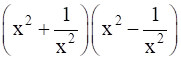Answer:using the identity (a + b) × (a – b) = a2 – b2here a = x2 and b =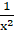⇒= (x2)2 -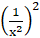Therefore= x4 -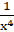Question 5.Use suitable identities to find the following products(1 + x) (1 + x)Answer:(1 + x) (1 + x) = (1 + x)2Using identity (a + b)2 = a2 + 2ab + b2Here a = 1 and b = x⇒ (1 + x) (1 + x) = 12 + 2(1)(x) + x2Therefore (1 + x) (1 + x) = 1 + 2x + x2Question 6.Evaluate the following products without actual multiplication.101 × 99Answer:101 can be written as (100 + 1) and99 can be written as (100 - 1)⇒ 101 × 99 = (100 + 1) × (100 - 1)using the identity (a + b) × (a – b) = a2 – b2here a = 100 and b = 1⇒ 101 × 99 = 1002 – 12⇒ 101 × 99 = 10000 – 1⇒ 101 × 99 = 9999Question 7.Evaluate the following products without actual multiplication.999 × 999Answer:999 can be written as (1000 – 1)⇒ 999 × 999 = (1000 – 1) × (1000 – 1)⇒ 999 × 999 = (1000 – 1)2Using identity (a – b)2 = a2 – 2ab + b2Here a = 1000 and b = 1⇒ 999 × 999 = 10002 – 2(1000)(1) + 12⇒ 999 × 999 = 1000000 – 2000 + 1⇒ 999 × 999 = 998000 + 1⇒ 999 × 999 = 998001Question 8.Evaluate the following products without actual multiplication.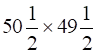Answer:⇒ 50=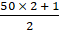and 49=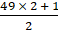⇒ 50=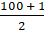and 49=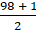⇒ 50=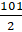and 49=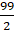⇒=×⇒=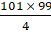Consider 101 × 99101 can be written as (100 + 1) and99 can be written as (100 - 1)⇒ 101 × 99 = (100 + 1) × (100 - 1)using the identity (a + b) × (a – b) = a2 – b2here a = 100 and b = 1⇒ 101 × 99 = 1002 – 12⇒ 101 × 99 = 10000 – 1⇒ 101 × 99 = 9999Therefore=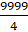Question 9.Evaluate the following products without actual multiplication.501 × 501Answer:501 can be written as (500 + 1)⇒ 501 × 501 = (500 + 1) × (500 + 1)⇒ 501 × 501 = (500 + 1)2⇒ 501 × 501 = (500 + 1) × (500 + 1)⇒ 501 × 501 = (500 + 1)2Using identity (a + b)2 = a2 + 2ab + b2Here a = 500 and b = 1⇒ 501 × 501 = 5002 + 2(500)(1) + 12⇒ 501 × 501 = 250000 + 1000 + 1⇒ 501 × 501 = 251001Question 10.Evaluate the following products without actual multiplication.30.5 × 29.5Answer:30.5 =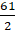and 29.5 =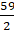⇒ 30.5 × 29.5 =×⇒ 30.5 × 29.5 =×⇒ 30.5 × 29.5 =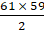…(i)Consider 61 × 5961 = (60 + 1)59 = (60 – 1)⇒ 61 × 59 = (60 + 1)(60 – 1)using the identity (a + b) × (a – b) = a2 – b2here a = 60 and b = 1⇒ 61 × 59 = 602 – 12⇒ 61 × 59 = 3600 – 1⇒ 61 × 59 = 3599From (i)⇒ 30.5 × 29.5 =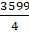Therefore 30.5 × 29.5 = 899.75Question 11.Factorise the following using appropriate identities.16x2 + 24xy + 9y2Answer:16x2 can be written as (4x)224xy can be written as 2(4x)(3y)9y2 can be written as (3y)2⇒ 16x2 + 24xy + 9y2 = (4x)2 + 2(4x)(3y) + (3y)2Using identity (a + b)2 = a2 + 2ab + b2Here a = 4x and b = 3y⇒ 16x2 + 24xy + 9y2 = (4x + 3y)2Therefore 16x2 + 24xy + 9y2 = (4x + 3y) (4x + 3y)Question 12.Factorise the following using appropriate identities.4y2 - 4y + 1Answer:4y2 can be written as (2y)24y can be written as 2(1)(2y)1 can be written as 12⇒ 4y2 - 4y + 1 = (2y)2 - 2(1)(2y) + 12Using identity (a - b)2 = a2 - 2ab + b2Here a = 2y and b = 1⇒ 4y2 - 4y + 1 = (2y - 1)2Therefore 4y2 - 4y + 1 = (2y - 1) (2y - 1)Question 13.Factorise the following using appropriate identities.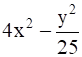Answer:4x2 can be written as (2x)2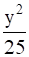can be written as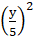⇒ 4x2 -= (2x)2 -using the identity (a + b) × (a – b) = a2 – b2here a = 2x and b =Therefore 4x2 -= (2x +) (2x -)Question 14.Factorise the following using appropriate identities.18a2 – 50Answer:Take out common factor 2⇒ 18a2 – 50 = 2 (9a2 - 25)Now9a2 can be written as (3a)225 can be written as 52⇒ 18a2 – 50 = 2 ((3a)2 – 52)using the identity (a + b) × (a – b) = a2 – b2here a = 3a and b = 5therefore 18a2 – 50 = 2 (3a + 5) (3a – 5)Question 15.Factorise the following using appropriate identities.x2 + 5x + 6Answer:Given is quadratic equation which can be factorised by splitting the middle term as shown⇒ x2 + 5x + 6 = x2 + 3x + 2x + 6= x (x + 3) + 2 (x + 3)= (x + 3) (x + 2)Therefore x2 + 5x + 6 = (x + 3) (x + 2)Question 16.Factorise the following using appropriate identities.3p2 - 24p + 36Answer:Take out common factor 3⇒ 3p2 - 24p + 36 = 3 (p2 – 8p + 12)Now splitting the middle term of quadratic p2 – 8p + 12 to factorise it⇒ 3p2 - 24p + 36 = 3 (p2 – 6p – 2p + 12)= 3 [p (p – 6) – 2 (p – 6)]= 3 (p – 2) (p – 6)Question 17.Expand each of the following, using suitable identities(x + 2y + 4z)2Answer:Using (a + b + c)2 = a2 + b2 + c2 + 2ab + 2bc + 2caHere a = x, b = 2y and c = 4z⇒ (x + 2y + 4z)2 = x2 + (2y)2 + (4z)2 + 2(x)(2y) + 2(2y)(4z) + 2(4z)(x)Therefore(x + 2y + 4z)2 = x2 + 4y2 + 16z2 + 4xy + 16yz + 8xzQuestion 18.Expand each of the following, using suitable identities(2a - 3b)3Answer:Using identity (x – y)3 = x3 - y3 – 3x2y + 3xy2Here x = 2a and y = 3b⇒ (2a - 3b)3 = (2a)3 – (3b)3 – 3(2a)2(3b) + 3(2a)(3b)2= 8a3 – 27b3 – 18a2b + 18ab2Therefore (2a - 3b)3 = 8a3 – 27b3 – 18a2b + 18ab2Question 19.Expand each of the following, using suitable identities(-2a + 5b - 3c)2Answer:(-2a + 5b - 3c)2 = [(-2a) + (5b) + (-3c)]2Using (x + y + z)2 = x2 + y2 + z2 + 2xy + 2yz + 2zxHere x = -2a, y = 5b and z = -3c⇒ (-2a + 5b - 3c)2 = (-2a)2 + (5b)2 + (-3c)2 + 2(-2a)(5b) + 2(5b)(-3c) + 2(-3c)(-2a)⇒ (-2a + 5b - 3c)2 = 4a2 + 25b2 + 9c2 + (-20ab) + (-30bc) + 12ac⇒ (-2a + 5b - 3c)2 = 4a2 + 25b2 + 9c2 - 20ab - 30bc + 12acTherefore(-2a + 5b - 3c)2 = 4a2 + 25b2 + 9c2 - 20ab - 30bc + 12acQuestion 20.Expand each of the following, using suitable identities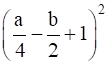Answer: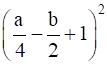= [+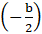+ 1]2Using (x + y + z)2 = x2 + y2 + z2 + 2xy + 2yz + 2zxHere x =, y =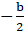and z = 1⇒=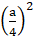+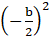+ 12 + 2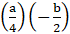+ 2(1) + 2(1)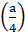⇒=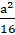+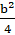+ 1 +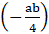+ (-b) +⇒=++ 1 -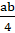– b +Therefore=++ 1 -– b +Question 21.Expand each of the following, using suitable identities(p + 1)3Answer:Using identity (x + y)3 = x3 + y3 + 3x2y + 3xy2Here x = p and y = 1⇒ (p + 1)3 = p3 + 13 + 3(p)2(1) + 3(p)(1)2= p3 + 13 + 3p2 + 3pTherefore (p + 1)3 = p3 + 13 + 3p2 + 3pQuestion 22.Expand each of the following, using suitable identities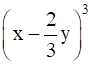Answer:Using identity (a – b)3 = a3 - b3 – 3a2b + 3ab2Here a = x and b =y⇒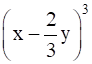= x3 -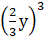– 3(x)2(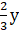) + 3(x)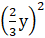⇒= x3 -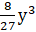– 2x2y +xy2Therefore= x3 -– 2x2y +xy2Question 23.Factorise25x2 + 16y2 + 4z2 - 40xy + 16yz - 20xzAnswer:25x2 + 16y2 + 4z2 - 40xy + 16yz - 20xz25x2 + 16y2 + 4z2 - 40xy + 16yz - 20xz = 25x2 + 16y2 + 4z2 + (-40xy) + 16yz + (-20xz)25x2 can be written as (-5x)216y2 can be written as (4y)24z2 can be written as (2z)2-40xy can be written as 2(-5x)(4y)16yz can be written as 2(4y)(2z)-20xz can be written as 2(-5x)(2z)⇒ 25x2 + 16y2 + 4z2 - 40xy + 16yz - 20xz = (-5x)2 + (4y)2 +(2z)2 + 2(-5x)(4y) + 2(4y)(2z) + 2(-5x)(2z) …(i)Using (a + b + c)2 = a2 + b2 + c2 + 2ab + 2bc + 2caComparing (-5x)2 + (4y)2 + (2z)2 + 2(-5x)(4y) + 2(4y)(2z) + 2(-5x)(2z) with a2 + b2 + c2 + 2ab + 2bc + 2ca we geta = -5x, b = 4y and c = 2ztherefore(-5x)2 + (4y)2 + (2z)2 + 2(-5x)(4y) + 2(4y)(2z) + 2(-5x)(2z) = (- 5x + 4y + 2z)2From (i)25x2 + 16y2 + 4z2 - 40xy + 16yz - 20xz = (- 5x + 4y + 2z)2Question 24.Factorise9a2 + 4b2 + 16c2 + 12ab - 16bc - 24caAnswer:9a2 + 4b2 + 16c2 + 12ab - 16bc - 24ca9a2 + 4b2 + 16c2 + 12ab - 16bc - 24ca = 9a2 + 4b2 + 16c2 + 12ab + (-16bc) + (-24ca)9a2 can be written as (3a)24b2 can be written as (2b)216c2 can be written as (-4c)212ab can be written as 2(3a)(2b)-16bc can be written as 2(2b)(-4c)-24ca can be written as 2(-4c)(3a)⇒ 9a2 + 4b2 + 16c2 + 12ab - 16bc - 24ca = (3a)2 + (2b)2 + (-4c)2 + 2(3a)(2b) + 2(2b)(-4c) + 2(-4c)(3a) …(i)Using (x + y + z)2 = x2 + y2 + z2 + 2xy + 2yz + 2zxComparing (3a)2 + (2b)2 + (-4c)2 + 2(3a)(2b) + 2(2b)(-4c) + 2(-4c)(3a) with x2 + y2 + z2 + 2xy + 2yz + 2zx we getx = 3a, y = 2b and z = -4ctherefore(3a)2 + (2b)2 + (-4c)2 + 2(3a)(2b) + 2(2b)(-4c) + 2(-4c)(3a) = (3a + 2b + (-4c))2From (i)9a2 + 4b2 + 16c2 + 12ab - 16bc - 24ca = (3a + 2b – 4c)2

PDF FILE TO YOUR EMAIL IMMEDIATELY PURCHASE NOTES & PAPER SOLUTION. @ Rs. 50/- each (GST extra)

HINDI ENTIRE PAPER SOLUTION

MARATHI PAPER SOLUTION

SSC MATHS I PAPER SOLUTION

SSC MATHS II PAPER SOLUTION

SSC SCIENCE I PAPER SOLUTION

SSC SCIENCE II PAPER SOLUTION

SSC ENGLISH PAPER SOLUTION

SSC & HSC ENGLISH WRITING SKILL

HSC ACCOUNTS NOTES

HSC OCM NOTES

HSC ECONOMICS NOTES

HSC SECRETARIAL PRACTICE NOTES

# 2019 Board Paper Solution

HSC ENGLISH SET A 2019 21st February, 2019

HSC ENGLISH SET B 2019 21st February, 2019

HSC ENGLISH SET C 2019 21st February, 2019

HSC ENGLISH SET D 2019 21st February, 2019

SECRETARIAL PRACTICE (S.P) 2019 25th February, 2019

HSC XII PHYSICS 2019 25th February, 2019

CHEMISTRY XII HSC SOLUTION 27th, February, 2019

OCM PAPER SOLUTION 2019 27th, February, 2019

HSC MATHS PAPER SOLUTION COMMERCE, 2nd March, 2019

HSC MATHS PAPER SOLUTION SCIENCE 2nd, March, 2019

SSC ENGLISH STD 10 5TH MARCH, 2019.

HSC XII ACCOUNTS 2019 6th March, 2019

HSC XII BIOLOGY 2019 6TH March, 2019

HSC XII ECONOMICS 9Th March 2019

SSC Maths I March 2019 Solution 10th Standard11th, March, 2019

SSC MATHS II MARCH 2019 SOLUTION 10TH STD.13th March, 2019

SSC SCIENCE I MARCH 2019 SOLUTION 10TH STD. 15th March, 2019.

SSC SCIENCE II MARCH 2019 SOLUTION 10TH STD. 18th March, 2019.

SSC SOCIAL SCIENCE I MARCH 2019 SOLUTION20th March, 2019

SSC SOCIAL SCIENCE II MARCH 2019 SOLUTION, 22nd March, 2019

XII CBSE - BOARD - MARCH - 2019 ENGLISH - QP + SOLUTIONS, 2nd March, 2019

# HSCMaharashtraBoardPapers2020

(Std 12th English Medium)

HSC ECONOMICS MARCH 2020

HSC OCM MARCH 2020

HSC ACCOUNTS MARCH 2020

HSC S.P. MARCH 2020

HSC ENGLISH MARCH 2020

HSC HINDI MARCH 2020

HSC MARATHI MARCH 2020

HSC MATHS MARCH 2020

# SSCMaharashtraBoardPapers2020

(Std 10th English Medium)

English MARCH 2020

HindI MARCH 2020

Hindi (Composite) MARCH 2020

Marathi MARCH 2020

Mathematics (Paper 1) MARCH 2020

Mathematics (Paper 2) MARCH 2020

Sanskrit MARCH 2020

Sanskrit (Composite) MARCH 2020

Science (Paper 1) MARCH 2020

Science (Paper 2)

Geography Model Set 1 2020-2021

MUST REMEMBER THINGS on the day of Exam

Are you prepared? for English Grammar in Board Exam.

Paper Presentation In Board Exam

How to Score Good Marks in SSC Board Exams

Tips To Score More Than 90% Marks In 12th Board Exam

How to write English exams?

How to prepare for board exam when less time is left

How to memorise what you learn for board exam

No. 1 Simple Hack, you can try out, in preparing for Board Exam

How to Study for CBSE Class 10 Board Exams Subject Wise Tips?

JEE Main 2020 Registration Process – Exam Pattern & Important Dates

NEET UG 2020 Registration Process Exam Pattern & Important Dates

How can One Prepare for two Competitive Exams at the same time?

8 Proven Tips to Handle Anxiety before Exams!